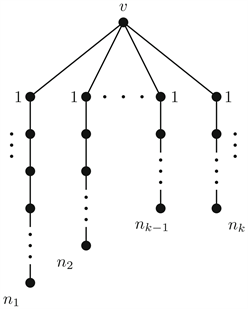﻿ 一类树的主特征值数目刻画

# 一类树的主特征值数目刻画Characterization of the Number of Main Eigenvalues of a Class of Trees

Abstract: The eigenvalues of the adjacency matrix of a graph are called the eigenvalues of a graph, the multi-set of all eigenvalues of a graph is called the spectrum of a graph. An eigenvalue of a graph is a main eigenvalue if its eigenspace is not orthogonal to the all-ones vector, the main eigenvalues are significant to the characterization of graphs and the properties of graphs. Characterizing graphs with k(2≤k≤n) number of main eigenvalues are a long-standing problem. In this paper, the lower bound of main eigenvalues of a class of trees is determined.

1. 引言

2. 预备知识

$k=1$ 时， ${\phi }_{2}\left(-x\right)=-x{\phi }_{1}\left(-x\right)-{\phi }_{0}\left(-x\right)=x{\phi }_{1}\left(x\right)-{\phi }_{0}\left(x\right)={\phi }_{2}\left(x\right)$

${\phi }_{3}\left(-x\right)=-x{\phi }_{2}\left(-x\right)-{\phi }_{1}\left(-x\right)=-x{\phi }_{2}\left(x\right)+{\phi }_{1}\left(x\right)=-{\phi }_{3}\left(x\right)$，成立。

$k=2$ 时， ${\phi }_{4}\left(-x\right)=-x{\phi }_{3}\left(-x\right)-{\phi }_{2}\left(-x\right)=x{\phi }_{3}\left(x\right)-{\phi }_{2}\left(x\right)={\phi }_{4}\left(x\right)$

${\phi }_{5}\left(-x\right)=-x{\phi }_{4}\left(-x\right)-{\phi }_{3}\left(-x\right)=-x{\phi }_{4}\left(x\right)+{\phi }_{3}\left(x\right)=-{\phi }_{5}\left(x\right)$，成立。Figure 1. $S\left({n}_{1},{n}_{2},{n}_{3},\cdots ,{n}_{k}\right)$

$Spec\left(S\left(1,1,m\right)\right)=\left\{2\mathrm{cos}\left(\frac{2k+1}{2m+4}\pi \right):k=0,1,\cdots ,m+1\right\}\cup \left\{0\right\}$

3. 一类星形树的主特征值数目

$\left\{\begin{array}{l}{x}_{3}=\lambda {x}_{1}\\ {x}_{3}=\lambda {x}_{2}\\ {x}_{1}+{x}_{2}+{x}_{4}=\lambda {x}_{3}\\ {x}_{3}+{x}_{5}=\lambda {x}_{4}\\ {x}_{4}+{x}_{6}=\lambda {x}_{5}\\ \cdots \\ {x}_{n-2}+{x}_{n}=\lambda {x}_{n-1}\\ {x}_{n-1}=\lambda {x}_{n}\end{array}$ (1)

${x}_{n}=0$，则代入(1)式可得：

$\left\{\begin{array}{l}{x}_{1}+{x}_{2}=0\\ {x}_{n}={x}_{n-1}={x}_{n-2}={x}_{n-3}=\cdots ={x}_{5}={x}_{4}={x}_{3}=0\end{array}$

$2\underset{i=1}{\overset{n}{\sum }}{x}_{i}-{x}_{1}-{x}_{2}+{x}_{3}-{x}_{n}=\lambda \underset{i=1}{\overset{n}{\sum }}{x}_{i}$

$\left(2-\lambda \right)\underset{i=1}{\overset{n}{\sum }}{x}_{i}={x}_{1}+{x}_{2}-{x}_{3}+{x}_{n}$

${x}_{1}+{x}_{2}-{x}_{3}+{x}_{n}=0$ (2)

$\left(2-\lambda \right){x}_{1}+1=0$ (3)

1) 当 $m$ 为偶数时， $n=m+3$ 为奇数，对于特征值 $±\lambda$，若对应 $\lambda$ 的特征向量为 $x={\left({x}_{1},{x}_{2},\cdots ,{x}_{n-1},1\right)}^{T}$，因为 $S\left(1,1,m\right)$ 为二部图，连接在中心点 $v$ 的两个一度点和 ${P}_{m}$ 中与 $v$ 的距离为奇数的顶点可划分为一个顶点集，则由推论2.8可得对应 $-\lambda$ 的特征向量为 $y={\left(-{x}_{1},-{x}_{2},{x}_{3},-{x}_{4},{x}_{5},\cdots ,{x}_{n-2},-{x}_{n-1},1\right)}^{\text{T}}$$x$$y$ 的第一个分量异号且不等于0，则 $±\lambda$ 至少有一个为主特征值，这就说明对于奇数个顶点的图 $S\left(1,1,m\right)$，其至少有 $\frac{m+3-1}{2}$ 个主特征值。

2) 当 $m$ 为奇数时， $n=m+3$ 为偶数，对于特征值 $±\lambda$，若对应 $\lambda$ 的特征向量为 $x={\left({x}_{1},{x}_{2},\cdots ,{x}_{n-1},1\right)}^{\text{T}}$，同理可得对应 $-\lambda$ 的特征向量为 $y={\left({x}_{1},{x}_{2},-{x}_{3},{x}_{4},-{x}_{5},\cdots ,-{x}_{n-2},{x}_{n-1},1\right)}^{\text{T}}$$x$$y$ 的第一个分量同号且不等于0，说明对于偶数个顶点的图 $S\left(1,1,m\right)$，其特征值 $±\lambda$ 同为主特征值(或非主特征值)。

${x}_{n}=1$ 代入(1)式并化简得：

$\left\{\begin{array}{l}{x}_{3}=\lambda {x}_{4}-{x}_{5}\\ {x}_{4}=\lambda {x}_{5}-{x}_{6}\\ \cdots \\ {x}_{n-2}=\lambda {x}_{n-1}-{x}_{n}\\ {x}_{n-1}=\lambda \\ {x}_{n}=1\end{array}$

$\left\{\begin{array}{l}{\phi }_{n-3}\left(\lambda \right)={x}_{3}\\ {\phi }_{n-4}\left(\lambda \right)={x}_{4}\\ \cdots \\ {\phi }_{2}\left(\lambda \right)={x}_{n-2}\\ {\phi }_{1}\left(\lambda \right)={x}_{n-1}\\ {\phi }_{0}\left(\lambda \right)={x}_{n}\end{array}$

${x}_{1}+{x}_{2}=\lambda {x}_{3}-{x}_{4}⇒{x}_{1}+{x}_{2}=\lambda {\phi }_{n-3}\left(\lambda \right)-{\phi }_{n-4}\left(\lambda \right)={\phi }_{n-2}\left( \lambda \right)$

${\phi }_{n}\left(x\right)$ 的奇偶性可得，

${\phi }_{0}\left(0\right)=1,{\phi }_{1}\left(0\right)=0,{\phi }_{2}\left(0\right)=-1,{\phi }_{3}\left(0\right)=0,{\phi }_{4}\left(0\right)=1,\cdots ,{\phi }_{4t}\left(0\right)=1,{\phi }_{4t+2}\left(0\right)=-1,\left(t=0,1,\cdots \right)$

 Cvetković, D. (1978) The Main Part of the Spectrum, Divisors and Switching of Graphs. Publications de l’Institut Mathématique (Beograd), 23, 31-38.

 Butler, G. and McKay, J. (1983) The Transitive Groups of Degree up to Eleven. Communications in Algebra, 11, 836-911.
https://doi.org/10.1080/00927878308822884

 Hagos, E.M. (2002) Some Results on Graph Spectra. Linear Algebra and Its Applications, 356, 103-111.
https://doi.org/10.1016/S0024-3795(02)00324-5

 Hayat, S., Koolen, J.H., Liu, F. and Qiao, Z. (2016) A Note on Graphs with Exactly Two Main Eigenvalues. Linear Algebra and Its Applications, 511, 318-327.
https://doi.org/10.1016/j.laa.2016.09.019

 Hou, Y.P., Tang, Z.K. and Shiu, W.C. (2012) Some Results on Graphs with Exactly Two Main Eigenvalues. Applied Mathematics Letters, 25, 1274-1278.
https://doi.org/10.1016/j.aml.2011.11.025

 Hou, Y.P. and Tian, F. (2006) Unicyclic Graphs with Exactly Two Main Eigenvalues. Applied Mathematics Letters, 19, 1143-1147.
https://doi.org/10.1016/j.aml.2005.11.025

 Hou, Y.P. and Zhou, H.Q. (2005) Trees with Exactly Two Main Eigenvalues. Journal of Natural Science of Hunan Normal University, 26, 1-3. (In Chinese)

 Lepović, M. (2001) Some Results on Graphs with Exactly Two Main Eigenvalues. Publikacija Elektrotehnikog Fakulteta Serija Matematika, 12, 68-84.

 Shi, L. (2009) On Graphs with Given Main Eigenvalues. Applied Mathematics Letters, 22, 1870-1874.
https://doi.org/10.1016/j.aml.2009.06.027

 Godsil, C. (2012) Controllable Subsets in Graphs. Annals of Com-binatorics, 16, 733-744.
https://doi.org/10.1007/s00026-012-0156-3

 O’Rourke, S. and Touri, B. (2016) On a Conjecture of Godsil Concerning Controllable Random Graphs. SIAM Journal on Control and Optimization, 54, 3347-3378.
https://doi.org/10.1137/15M1049622

 高随祥. 图论与网络流理论[M]. 北京: 高等教育出版社, 2009.

 Biggs, N. Algebraic Graph Theory [M]. 北京: 世界图书出版公司, 2004.

 Oboudi, R.M. (2018) On the Eigenvalues and Spectral Radius of Starlike Trees. Aequationes Mathematicae, 92, 683-694.
https://doi.org/10.1007/s00010-017-0533-4

Top